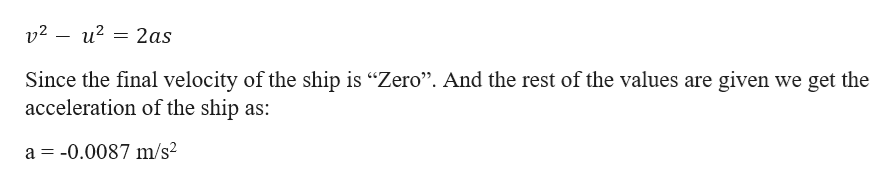Modern oil tankers weigh over a half-million tons and have lengths of up to one-fourth mile. Such massive ships require a distance of 4.5 km (about 2.8 mi) and a time of 17 min to come to a stop from a top speed of 32 km/h.(a) What is the magnitude of such a ship's average acceleration in m/s2 in coming to a stop?(b) What is the magnitude of the ship's average velocity in m/s?

Question

Modern oil tankers weigh over a half-million tons and have lengths of up to one-fourth mile. Such massive ships require a distance of 4.5 km (about 2.8 mi) and a time of 17 min to come to a stop from a top speed of 32 km/h.

(a) What is the magnitude of such a ship's average acceleration in m/s2 in coming to a stop?

(b) What is the magnitude of the ship's average velocity in m/s?

Step 1

Given information:

Distance required to come to rest (s) = 4.5 km = 4500 m.

Time required to stop (t) = 17 min = 17 × 60 s = 1020 s.

Top speed of the ship (u) = 32 kmph = 32 × (5/18) m/s = 8.8 m/s

Step 2

From 3rd equation of motion we can find the acceleration (which is retardation) as follows:help_outlineImage Transcriptionclosev2 u2 2as Since the final velocity of the ship is "Zero". And the rest of the values are given we get the acceleration of the ship as: a 0.0087 m/s2 fullscreen
Step 3

The magnitude of average velocity (Vavg) is total dista...

Want to see the full answer?

See Solution

Want to see this answer and more?

Our solutions are written by experts, many with advanced degrees, and available 24/7

See Solution
Tagged in

Physics